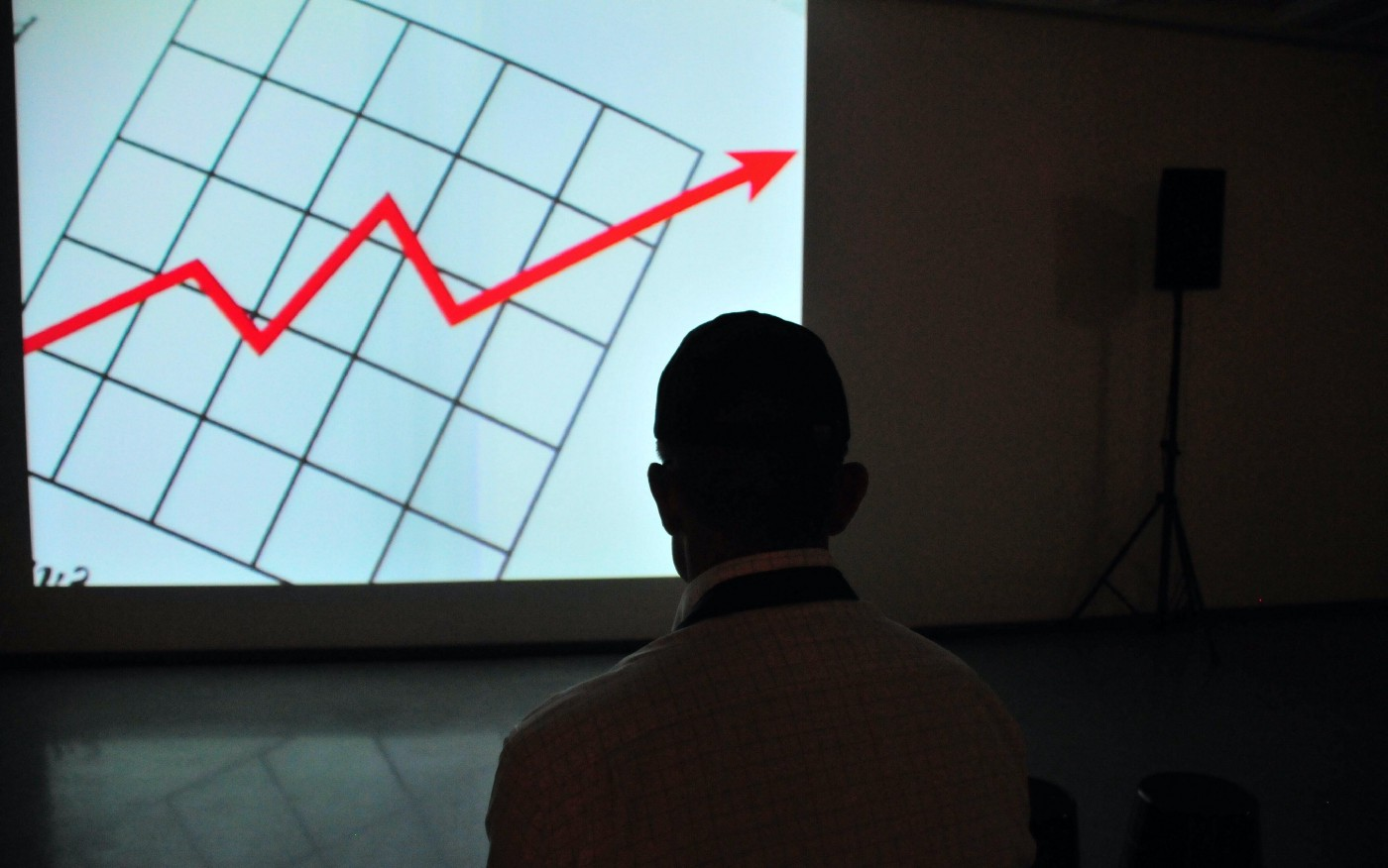Tutorials
April 13, 2022

# How to test ML models? #3 📈 Numerical data drift

Testing the drift of numerical feature distribution is essential in AI. Here are the key metrics you can use to detect it.Jean-Marie John-Mathews, Ph.D.Numerical data drift

Wasserstein metrics, Earthmover distance, Kolmogorov test… Drift for numerical features in Machine Learning can be tested using many methods. How to choose the right one?

Testing drifts of ML feature distribution is essential in AI. It enables you to make sure your model is still valid by checking if data in inference time is close to the data used for training the model. Data drift measures the difference in statistical distribution between a reference data, often the training set, and a “current” dataset, such as the test set or a batch of examples in inference time.

In our last post, we addressed how to compute tests for drift detection of categorical features in Machine Learning. In this post, we rather deal with numerical features. Contrary to categorical features, distribution for numerical features is a bit harder to compute, hence the need for different metrics. We give the definition, formula, and interpretation of the main numerical drift metrics between two datasets.

Notation:

• The cumulative distribution function of a variable evaluated at x is the proportion of the data points that are below x.
• We note F_R as the cumulative distributive function of the feature inside the reference dataset (usually the train set) and F_C as the one for the current data set (usually the test set at training time or a batch of examples in production).

## Earth mover’s distance

One of the most used metrics for numerical feature drift in ML is the first Wasserstein metric also called the earthmover distance. The mathematical formulation of this metrics is quite straightforward, it’s the difference in the cumulative distribution function between the current and reference datasets.

Intuitively, if each distribution is viewed as piles of soil (earth), the earthmover metric is the minimum “cost” of turning one pile into the other. It is assumed to be the amount of earth that needs to be moved times the mean distance it has to be moved.

In the code above, we use the Wasserstein distance implemented by sci-kit learn. It computes the integral as a weighted sum of all the values taken by the numerical feature in the two datasets (for technical details, see the Dirac function in cdf_distance). We normalised both distributions so that the Eartmover’s distance is between 0 and 1.

While the earth mover’s distance is easy to interpret, it does not provide any threshold that could be used for testing purposes. Traditional statistical tests are more convenient because they provide a threshold for decision-making. Let’s see how it works with the Kolmogorov-Smirnov test.

## The Kolmogorov-Smirnov test

The two-samples Kolmogorov-Smirnov by Andrey Kolmogorov and Nikolai Smirnov is a very common statistical test. It enables to answer whether two sets of samples are drawn from the same (but unknown) probability distribution. It’s the highest value of the difference in cumulative distribution functions between current and reference datasets. Formally, it’s defined by :

Statistically, when the number of datapoints is sufficiently large, D_n follows a Kolmogorov law. As a result, if D_n is above the 95% quantile of the Kolmogorov distribution, one can reject the fact that the features R and C are coming from the same distribution. We then have a rigorous statistical basis for testing the drift between C and D.

Because of the upper limit (sup), the KS metric is much more sensitive to local changes compared to the Earthmovers distance. For example, suppose that your test set has many datapoints from people aged exactly 36 years. Let’s also suppose that apart from 36 years old people, all the other ages have the exact distribution between train and test sets. In that case, KS metric for the age feature will be very large and the KS drift test will certainly be rejected. KS metric is then sensitive to local differences in distribution functions.

If only the shape of the distribution is essential, Earthmover’s test might be more appropriate for numerical drift testing. Furthermore, the statistical basis of KS testing is only available when the number of datapoints is sufficiently large. Otherwise, the benefits of the threshold are no longer valid.

Below is an implementation code of the Kolmogorov-Smirnov test in Machine Learning.

## Conclusion

While different ways exist to test the drift of numerical features in Machine learning, there is no best method. On the one hand, Earthmover’s drift testing offers a straightforward way to interpret drift between two numerical features. On the other hand, Kolmogorov-Smirnov provides a testing method based on probabilistic theory. But each of these methods has its downsides. It depends on what you mean by drifts, given your domain requirements!

At Giskard, we provide open-source resources for tuning these ML testing methods and integrating them into your model.

Check our Github!

## References

• “Wasserstein metric”, Wikipedia
• Ramdas, Garcia, Cuturi “On Wasserstein Two Sample Testing and Related Families of Nonparametric Tests” (2015). Arxiv link
• Hodges, J.L. Jr., “The Significance Probability of the Smirnov Two-Sample Test,” Arkiv fiur Matematik, 3, №43 (1958), 469–86. Article link

{{richtext-style-text-line}}